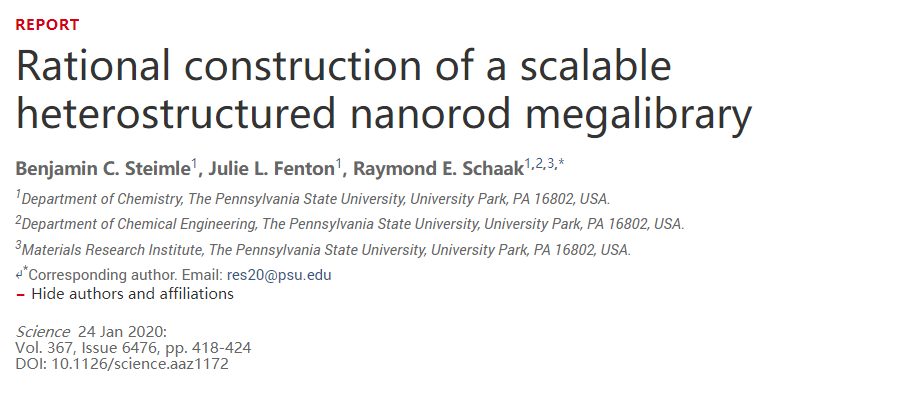0%

# 最近更新

• 新增项目板块，已更新期刊信息可视化
• 新域名https://mozheyang.com/已同时启用。
• 评论功能目前仍处于并可能长期处于测试状态。

# 热门博客

$$Z'(\omega) = \frac{1}{\pi} P \int^{\infty}_{-\infty} \frac{Z''(\omega')}{\omega'-\omega} \text{d}\omega'$$
$$Z''(\omega) = -\frac{1}{\pi} P \int^{\infty}_{-\infty} \frac{Z'(\omega')}{\omega'-\omega} \text{d}\omega'$$# 交流阻抗谱学的优势在哪？